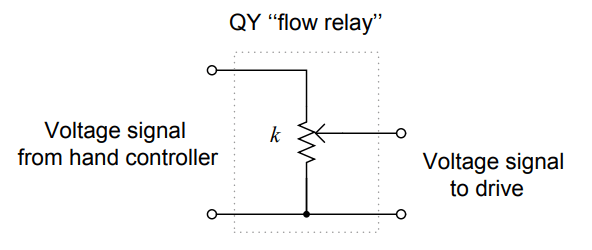# Problem on Weigh feeder

Weigh feeders are conveyor belts equipped with load cells to measure the weight of material on a section of the belt, and variable-speed motor drives to adjust the rate at which the belts feed material into a process.

When different solid materials are mixed together to make a blend, the weigh feeders must be connected together in a ratio configuration to maintain the desired proportion(s):

## Weigh feederThis particular system mixes salt, yeast, and flour together to make dry bread mix in a large factory. The “hand controller” is nothing more than an operator station where a person can set a voltage signal value, in this case, to be sent to a series of multiplier relays (QY) to tell each variable-speed motor drive how fast to turn.

Like many variable-speed motor drives, these units accept a 0 to 10 volt DC speed signal as an alternative to the standard 4-20 mA DC signal used in loop-powered instrumentation. This makes the multiplier relays (QY) nothing more than potentiometers:Given the following full-scale weigh feeder capacities (pounds per minute at full 10 volt speed signal), determine the actual ratios of salt to yeast to flour when the three potentiometers are set to the following values:

Weigh feeder capacities:

• Flour weigh feeder: 100 pounds per minute
• Yeast weigh feeder: 1.5 pounds per minute
• Salt weigh feeder: 0.5 pounds per minute

Potentiometer settings:

• kflour = 50%
• kyeast = 60%
• ksalt = 45%

Also, determine the total mix flow rate in pounds per minute with a hand controller setting of 70%.

Flour ﬂow ratio = 97.8% of total ﬂow

Yeast ﬂow ratio = 1.76% of total ﬂow

Salt ﬂow ratio = 0.44% of total ﬂow

Total mix ﬂow rate at HC setting of 70% = 35.7875 pounds per minute

1.